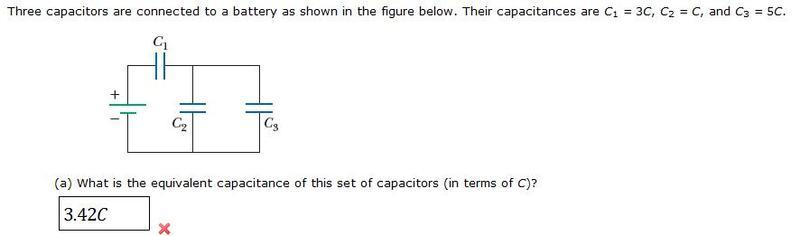# Equivalent Capacitance of a set of Capacitors

NotCarlSagan

## Homework Statement## Homework Equations

1/Ceq= 1/C1 + 1/C2

## The Attempt at a Solution

1/C13eq= 1/3C1 + 1/5C3 = 8C/3 = 2.67C

1/C12eq= (3C x C)/(3C + C) = 0.75C

Ceqtotal = 2.67C + 0.75C = 3.42C

I think the series circuit is throwing me off?

voko
Consider the combination of C2 and C3 as a single capacitor in series with C1.